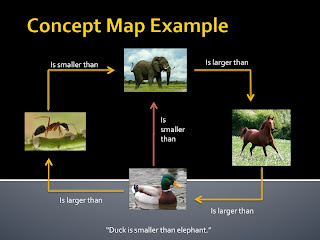## Sunday, August 29, 2010

### Metric System Concept Map

A few days ago, students completed what could be considered my best processing activity ever planned to date. It was creative, rigorous, and required deep understanding of the topic.

On the right side of their notebook, students recorded notes on relationships between metric units. We practiced with conversions together (if a seat belt is 3 meters long, how many centimeters? how many millimeters?). Students need to be comfortable converting between measured units in the lab and then units required for formulas. They also need to be grounded in a firm understanding of the metric system, and be able to articulate larger than/smaller than comparisons.

I first introduced the assignment with a simple concept map, to illustrate what a concept map is.The left side processing activity was a concept map with four boxes: kilometer, meter, centimeter, millimeter. Students connected the boxes with ten arrows total: six arrows with larger than/smaller than comparisons, four arrows with conversions (for example, 3 meters = 300 centimeters).